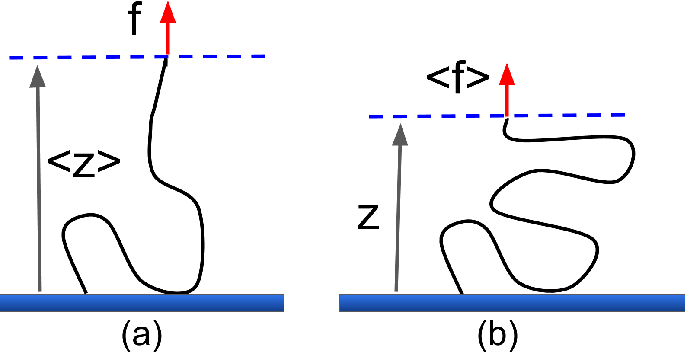## 接枝高斯链系综不等价性- 阅读剩余部分 -

## 如何编译物理学报latex模板？

1. 用TeX Live编译不了，用ctex可以
2. 用ctex编译报错。解决方法：删掉 aappss.sty文件第一行的"i"字母，如下图所示。3. 打开tex文件乱码，方法将文件用记事本或notepad等打开复制再粘贴在tex文件中。
以上参考了：物理学报latex模板不能编译问题

- 阅读剩余部分 -

## 自由空间中的高斯连系综等价性- 阅读剩余部分 -

## 聚电解质分子刷的标度理论- 阅读剩余部分 -

## 聚电解质刷浸没于纳米粒子溶液中- 阅读剩余部分 -

## 纳米粒子添加物对高分子凝胶体积转变的影响• $\phi$：凝胶体积分数
• $\phi_0$：凝胶参考态体积分数
• $N$：凝胶内支链长度
• $\psi$：纳米粒子体积分数
• $\chi_{ij}$：组分$i$与$j$之间的弗洛里-哈金斯参数

- 阅读剩余部分 -

## 微凝胶的泊松-玻尔兹曼-弗洛里理论- 阅读剩余部分 -

## Emden-Lane边值问题变分迭代法

Emden-Lane边值问题：

\begin{equation} \begin{split} & u''(x)+\frac{\alpha}{x}u'(x)=f(x,u) \\ & u'(0)=0, au(1)+bu'(1)=c \end{split} \label{Emden-Lane} \end{equation}

\begin{equation} u_{n+1}(x)=u_n(x)+\int_0^x\lambda(s)\left( u_n''(s)+\frac{\alpha}{s}u_n'(s)-f(s,\widetilde{u}_n) \right) \mathrm ds \label{correctionalfunc} \end{equation}

- 阅读剩余部分 -